# Parallel DC Circuits Practice Worksheet With Answers

## Basic Electricity

• #### Question 1

In this circuit, three resistors receive the same amount of voltage (24 volts) from a single source. Calculate the amount of current “drawn” by each resistor, as well as the amount of power dissipated by each resistor:• #### Question 2

What will happen to the brightness of the light bulb if the switch in this circuit is suddenly closed?• #### Question 3

 Don’t just sit there! Build something!!

Learning to mathematically analyze circuits requires much study and practice. Typically, students practice by working through lots of sample problems and checking their answers against those provided by the textbook or the instructor. While this is good, there is a much better way.

You will learn much more by actually building and analyzing real circuits, letting your test equipment provide the “answers” instead of a book or another person. For successful circuit-building exercises, follow these steps:

1. Carefully measure and record all component values prior to circuit construction.
2. Draw the schematic diagram for the circuit to be analyzed.
3. Carefully build this circuit on a breadboard or other convenient medium.
4. Check the accuracy of the circuit’s construction, following each wire to each connection point, and verifying these elements one-by-one on the diagram.
5. Mathematically analyze the circuit, solving for all values of voltage, current, etc.
6. Carefully measure those quantities, to verify the accuracy of your analysis.
7. If there are any substantial errors (greater than a few percent), carefully check your circuit’s construction against the diagram, then carefully re-calculate the values and re-measure.

Avoid very high and very low resistor values, to avoid measurement errors caused by meter “loading”. I recommend resistors between 1 kΩ and 100 kΩ, unless, of course, the purpose of the circuit is to illustrate the effects of meter loading!

One way you can save time and reduce the possibility of error is to begin with a very simple circuit and incrementally add components to increase its complexity after each analysis, rather than building a whole new circuit for each practice problem. Another time-saving technique is to re-use the same components in a variety of different circuit configurations. This way, you won’t have to measure any component’s value more than once.

• #### Question 4

The equation for calculating total resistance in a parallel circuit (for any number of parallel resistances) is sometimes written like this:

$$R_{total} = (R_1^{-1} + R_2^{-1} + ...R_n^{-1})^{-1}$$

Re-write this equation in such a way that it no longer contains any exponents.

• #### Question 5

Manipulate this equation to solve for resistor value R1, given the values of R2 and Rparallel:

$$R_{parallel} = \frac {R_1R_2}{R_1+R_2}$$

Then, give an example of a practical situation where you might use this new equation.

• #### Question 6

The formula for calculating total resistance of three parallel-connected resistors is as follows:

$$R = \frac {1}{\frac{1}{R_1}+\frac{1}{R_2}+\frac{1}{R_3}}$$

Algebraically manipulate this equation to solve for one of the parallel resistances (R1) in terms of the other two parallel resistances (R2 and R3) and the total resistance (R). In other words, write a formula that solves for R1 in terms of all the other variables.

• #### Question 7

Identify which of these circuits is a parallel circuit (there may be more than one shown!):• #### Question 8

Determine the amount of voltage impressed across each resistor in this circuit:Hint: locate all the points in this circuit that are electrically common to one another!

• #### Question 9

According to Ohm’s Law, how much current goes through each of the two resistors in this circuit?Draw the paths of all currents in this circuit.

• #### Question 10

Qualitatively compare the voltage and current for each of the three light bulbs in this circuit (assume the three light bulbs are absolutely identical):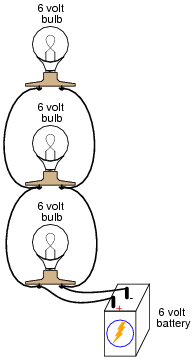• #### Question 11

Calculate the total amount of current that the battery must supply to this parallel circuit:Now, using Ohm’s Law, calculate total resistance (Rtotal) from total (source) voltage Vtotal and total (source) current Itotal.

• #### Question 12

Suppose I connect two resistors in parallel with one another, like this:How much electrical resistance would you expect an ohmmeter to indicate if it were connected across the combination of these two parallel-connected resistors?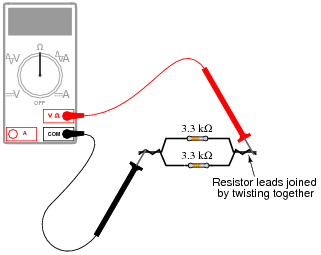Explain the reasoning behind your answer, and try to formulate a generalization for all combinations of parallel resistances.

• #### Question 13

There are two well-known formulae for calculating the total resistance of parallel-connected resistances. One of these works only for two resistances, while the other works for any number of parallel resistances. Write these two formulae, and give examples of their use.

• #### Question 14

A quantity often useful in electric circuit analysis is conductance, defined as the reciprocal of resistance:

$$G= \frac{1}{R}$$

In a series circuit, resistance increases and conductance decreases with the addition of more resistors: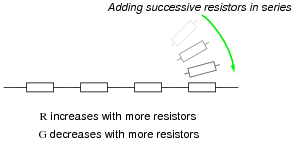Describe what happens to total resistance and total conductance with the addition of parallel resistors: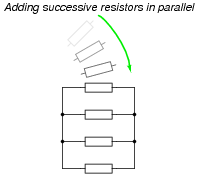• #### Question 15

Explain, step by step, how to calculate the amount of current (I) that will go through each resistor in this parallel circuit, and also the voltage (V) dropped by each resistor:• #### Question 16

Complete the table of values for this circuit:• #### Question 17

Complete the table of values for this circuit:• #### Question 18

In a parallel circuit, certain general rules may be stated with regard to quantities of voltage, current, resistance, and power. Express these rules, using your own words:

“In a parallel circuit, voltage . . .”

“In a parallel circuit, current . . .”

“In a parallel circuit, resistance . . .”

“In a parallel circuit, power . . .”

For each of these rules, explain why it is true.

• #### Question 19

What will happen in this circuit as the switches are sequentially turned on, starting with switch number 1 and ending with switch number 3?Describe how the successive closure of these three switches will impact:

• The voltage drop across each resistor
• The current through each resistor
• The total amount of current drawn from the battery
• The total amount of circuit resistance “seen” by the battery
• #### Question 20

The circuit shown here is commonly referred to as a current divider. Calculate the voltage dropped across each resistor, the current drawn by each resistor, and the total amount of electrical resistance ßeen” by the 9-volt battery: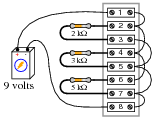• Current through the 2 kΩ resistor =
• Current through the 3 kΩ resistor =
• Current through the 5 kΩ resistor =
• Voltage across each resistor =
• Rtotal =

Can you think of any practical applications for a circuit such as this?

• #### Question 21

There is a simple equation that gives the equivalent resistance of two resistances connected in parallel. Write this equation.

Secondly, apply this two-resistance equation to the solution for total resistance in this three-resistor network:No, this is not a “trick” question! There is a way to apply a two-resistance equation to solve for three resistances connected in parallel.

• #### Question 22

Suppose you needed a resistance equal to precisely 235 Ω for the construction of a precision electrical meter circuit. The only resistors available to you are two 1 kΩ resistors, one 500 Ω resistor, and a rheostat variable between 600 and 1000 ohms. Design a parallel resistor network using any combination of these components that will yield a total resistance of 235 Ω. If you use the rheostat in your design, specify its resistance setting.

• #### Question 23

Draw the connecting wires on this terminal strip so that the three light bulbs are wired in parallel with each other and with the battery.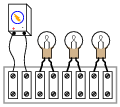• #### Question 24

Choose two resistor values such that one resistor passes 25% of the total current, while the other resistor passes 75% of the total current: